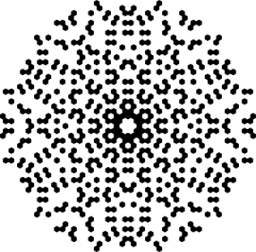# Twenty-five

How many are three-digit natural numbers divisible by 25?

n =  36

### Step-by-step explanation:Did you find an error or inaccuracy? Feel free to write us. Thank you!Tips to related online calculators
Do you want to perform natural numbers division - find the quotient and remainder?
Would you like to compute count of combinations?

## Related math problems and questions:

• Divisible by nineHow many three-digit natural numbers in total are divisible without a remainder by the number 9?
• 7 digit numberIf 3c54d10 is divisible by 330, what is the sum of c and d?
• How many 4How many four-digit numbers that are divisible by ten can be created from the numbers 3, 5, 7, 8, 9, 0 such no digits repeats?
• Three-digit integersHow many three-digit natural numbers exist that do not contain zero and are divisible by five?
• Five-digit numberAnna thinks of a five-digit number that is not divisible by three or four. If he increments each digit by one, it gets a five-digit number that is divisible by three. If he reduces each digit by one, he gets a five-digit number divisible by four. If it sw
• Four-digit numbersHow many four-digit numbers can we make from the numbers 2, 6, 3, 5, 1 and 9 if the numbers in the number cannot be repeated?
• Inverted nineIn the hotel Inverted Nine, each hotel room number is divisible by 6. How many rooms can we count with the three-digit number registered by digits 1,8,7,4,9?
• Three digits numberFrom the numbers 1, 2, 3, 4, 5 create three-digit numbers that digits not repeat and number is divisible by 2. How many numbers are there?
• No. of divisorsHow many different divisors have number 13 4 * 2 4?
• Three-digit numbersWe have digits 0,1,4,7 that cannot be repeated. How many three-digit numbers can we write from them? You can help by listing all the numbers.
• What are 3What are the two digits which when inserted in the blank spaces will make 234 _ _ divisible by 8?
• Divisible by fiveHow many different three-digit numbers divisible by five can we create from the digits 2, 4, 5? The digits can be repeated in the created number.
• NumbersDetermine the number of all positive integers less than 4183444 if each is divisible by 29, 7, 17. What is its sum?
• Prime divisorsFind 2/3 of the sum's ratio and the product of all prime divisors of the number 120.
• Three-digitHow many do three-digit natural numbers not have the number 7?
• A number 5A number is divisible by 24, 25, and 120 if it is increased by 20. Find the number.
• Three digits numberHow many are three-digit integers such that they no digit repeats?